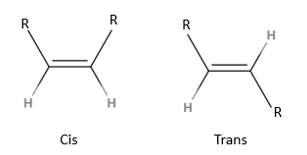# In a HWE reaction, a 1, 2-disubstituted alkene was formed in which the olefinic protons exhibited...

## Question:

In a HWE reaction, a 1, 2-disubstituted alkene was formed in which the olefinic protons exhibited a vicinal coupling of 9.0 Hz in the 1H NMR. What is the stereochemistry of the double bond?

## Proton NMR couplings

The interaction or coupling between two non-equivalent hydrogens in a proton NMR spectrum can be expressed in terms of a coupling constant (J). The value of J can be calculated by subtracting the values of the chemical shifts of two adjacent peaks in a multiplet (bigger with the smaller). The value is always expressed in terms of Hz, so often the ppm values of the chemicals shifts needs to be converted.

The product in this Horner Wadsworth Emmons (HWE) reaction is a 1,2-disubstituted alkene.

Either the cis or trans stereochemistry can be formed as shown in the image.Trans coupling is normally bigger than cis coupling on a proton NMR.

For trans configuration, the value of J (coupling constant) is between 11 - 19 Hz, while it is between 5 and 14 Hz for cis.

Based on this information we can assume a cis configuration since a J value of 9 Hz is mentioned in the question.How to Read NMR Spectra of Organic Compounds

from Organic Chemistry: Help & Review

Chapter 2 / Lesson 2
17K

Support